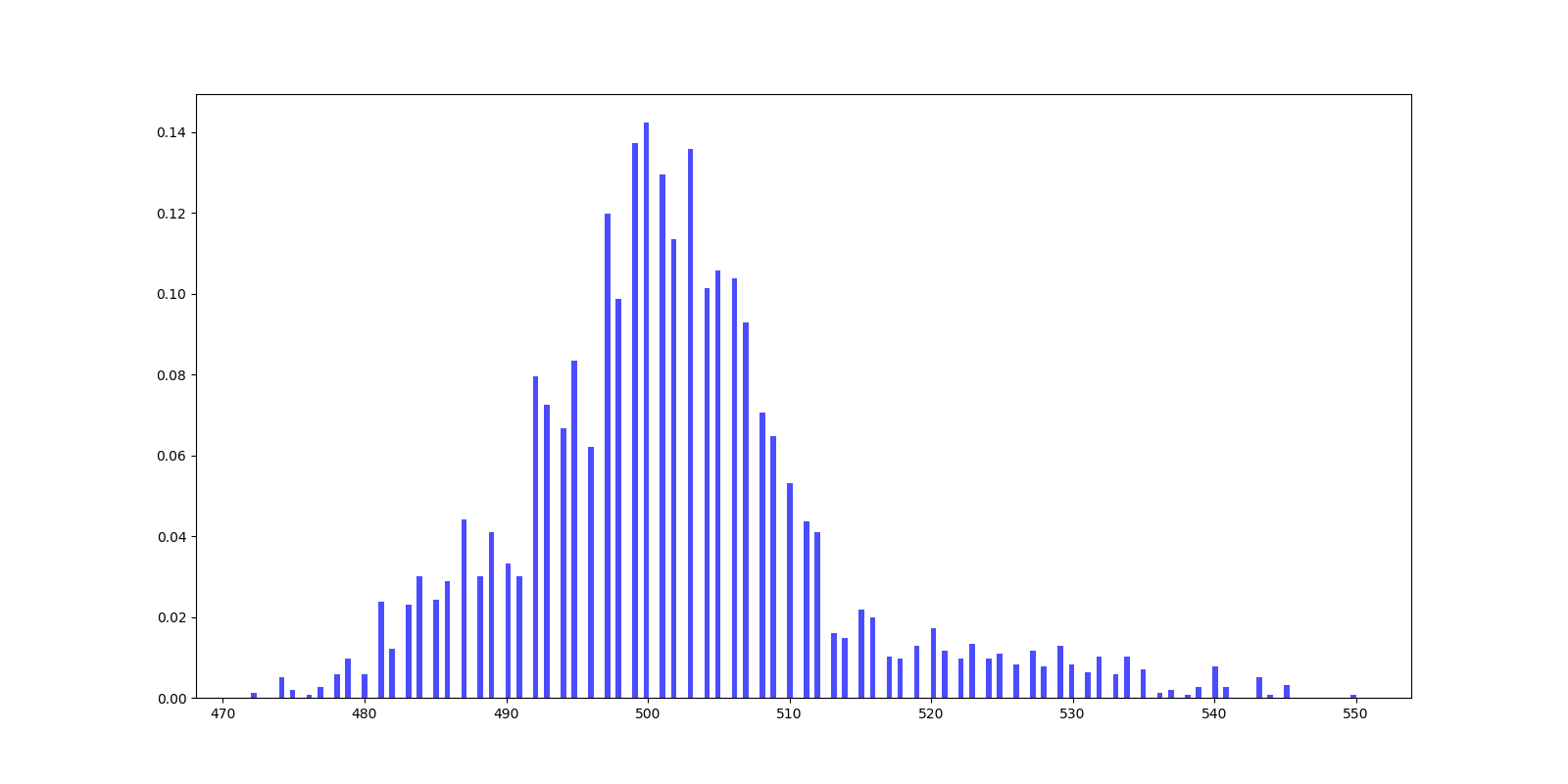2019.９.24

# ３天半的建模过程

第二天20号，我们开始仔细分析题目要求，分析所给数据的特征。下午师兄就开始讨论如何使用Tensorflow机器学习框架实现题目的要求，由于我只是对机器学习大概了解，还没有动手构建模型的编码能力，基本处于划水状态，经过题目思路的讨论，确定了工作分配。晚上师兄们就开始使用Tensorflow构建模型，我来翻阅资料提取特征，A题必须先上传模型，有跑分，才有资格评选论文。所以师兄的压力特别大，中途由于部署模型的困难，结果过于拟合的困难，几乎快要奔溃，但是师兄没有放弃，一直调试到夜里3点终于部署成功。
第三天21号，早上出打榜结果，我们排名靠前。但是还有好多队伍还没有提交，这不是最后的结果。虽然我们的模型部署成功，但是比较简单，还没有调优，我们只是使用了3个特征，准确的来说只有一个距离特征，所以训练出来的模型的效果不是很好，但是由于时间紧迫，我们决定先写论文，最后再优化。下午我们讨论了论文的整体思路，我先定了大概格式和目录，师兄开始写关键的主体内容，我来把之前数据的分析结果可视化（说来惭愧，整个建模就做了这一点点贡献），写论文的整个过程，真的非常痛苦，一直改到3点，大师兄实在太累了，我和二师兄开始修改，改到4点我也坚持不住了，就只有二师兄一个人在改了，到5点半我睡醒了，发现是大师兄已经醒了在改论文，二师兄休息了。
就这样到了6点，太阳出来了，我们的论文终于修改好了。但是这个时候我们更新打榜排名，我们发现我们的排名下降了好多。这个时候我们快放弃了，心里想，算了回去睡觉了，拿不到名次就算了吧，至少努力过了。但是二师兄，坚持利用好最后的5个小时开始调模型，我也帮不了什么忙，帮大师兄二师兄加油打气，最后一直到12点，又提交了几次模型，结果不是很好，但是我们互相鼓励，相信结果不会太差，就这样3天半的建模比赛结束了。

# 最终的论文摘要

针对问题一，要求参照Cost 231-Hata和给出的样例数据设计合适的特征。在设计特征时，本文从实际问题出发，考虑到5G基站发射机较以往的发射机发射频率更高的问题，调整Cost 231-Hata部分参数，以达到适应5G网络的特性。此外根据样例数据以及现实生活中信号传输的特性设计了7种简单特征，如水平距离、天线水平角夹角等。然后结合Cost 231-Hata和7种简单特征组合成7种复杂特征，总计14种特征。
针对问题二，要求对问题一设计的特征从是否发散、与目标的相关性等方面判断特征是否合适，从而为机器学习模型选择有效的特征。为此，本文选择了方差来判断特征的离散程度。另外选择了皮尔逊系数和互信息来判断特征的相关性。随后将判断的结果量化、排序并展示在表中。
针对问题三，要求使用参赛队伍自身设计的特征集和赛题提供的训练数据集，设计合适的AI模型来预测RSRP。为此，本文根据问题的特点设计了神经网络模型。在设计网络时使用了对称的编码解码结构，将16维的特征逐层编码到1024维，再逐渐解码至1维，以增强网络的表达能力。此外，对于要求弱覆盖识别率PCRR达到20%以上的问题，使用了弱覆盖信号数据加权以及减少强覆盖信号训练数据的数量，来增强神将网络对弱覆盖信号数据的学习偏好。最后本文对PCRR度量进行了修改，使其作为损失函数来优化网络。

# 建模中我的工作

1. 对第一个小区数据使用Cost 231-Hata和最终结果进行对比
import pandas as pd
import numpy as np
import matplotlib.pyplot as plt

np.set_printoptions(suppress=True)
col_name = train_data.columns.tolist()

def hata_feature(CX, CY, X, Y, CAltitude, CHeight, Altitude, Frequency, Power):
dis = np.sqrt((CX - X) ** 2 + (CY - Y) ** 2) / 1000
hb = CAltitude + CHeight - Altitude
cm = 3
pl = 46.3 + 83.9 * np.log(Frequency) - 13.82 * np.log(hb) - 0 + (44.9 - 6.55 * np.log(hb)) * np.log(dis) + cm
RSRP = Power - pl
return RSRP

RSRP = np.array(train_data[col_name].tolist())
CX = np.array(train_data[col_name].tolist())
CY = np.array(train_data[col_name].tolist())
X = np.array(train_data[col_name].tolist())
Y = np.array(train_data[col_name].tolist())
CHeight = np.array(train_data[col_name].tolist())
CAltitude = np.array(train_data[col_name].tolist())
Altitude = np.array(train_data[col_name].tolist())
Frequency = np.array(train_data[col_name].tolist())
Power = np.array(train_data[col_name].tolist())
rsrp = hata_feature(CX, CY, X, Y, CAltitude, CHeight, Altitude, Frequency, Power)

for i in range(len(rsrp)):
print(rsrp[i], RSRP[i])

1. 比较特征是否发散，判断对最终预测结果的影响
import pandas as pd
import numpy as np
import matplotlib.pyplot as plt
# %matplotlib inline

np.set_printoptions(suppress=True)
col_name = train_data.columns.tolist()

def lost_feature(CX, CY, X, Y, CAltitude, CHeight, Altitude, Frequency):
dis = np.sqrt((CX - X) ** 2 + (CY - Y) ** 2)
hb = (CAltitude + CHeight - Altitude)
d = np.sqrt(dis ** 2 + hb ** 2) / 1000
lost = 42.6 + 26 * np.log(d) + 20* np.log(Frequency)
# RSRP = Power - pl
return lost

RSRP = np.array(train_data[col_name].tolist())
CX = np.array(train_data[col_name].tolist())
CY = np.array(train_data[col_name].tolist())
X = np.array(train_data[col_name].tolist())
Y = np.array(train_data[col_name].tolist())
BH = np.array(train_data[col_name].tolist())
dis = np.sqrt((CX - X) ** 2 + (CY - Y) ** 2)
CHeight = np.array(train_data[col_name].tolist())
CAltitude = np.array(train_data[col_name].tolist())
Altitude = np.array(train_data[col_name].tolist())

Frequency = np.array(train_data[col_name].tolist())
Power = np.array(train_data[col_name].tolist())
lost = lost_feature(CX, CY, X, Y, CAltitude, CHeight, Altitude, Frequency)

# lost是否发散
n=1024
X=lost
Y=RSRP
T=np.arctan2(RSRP,lost)			#设置颜色
lost_var = np.var(lost)
print(lost_var)
plt.scatter(X,Y,s=5,c=T,alpha=1)	#　s：表示大小，alpha:表示透明度
plt.show()

# Frequency是否发散
n=1024
X=Frequency
Y=RSRP
T=np.arctan2(RSRP,Frequency)			#设置颜色
Frequency_var = np.var(Frequency)
print(Frequency_var)
plt.scatter(X,Y,s=5,c=T,alpha=1)	#　s：表示大小，alpha:表示透明度
plt.show()

# Building Height
X=BH
Y=RSRP
T=np.arctan2(RSRP,BH)			#设置颜色
BH_var = np.var(BH)
print(BH_var)
plt.scatter(X,Y,s=5,c=T,alpha=1)	#　s：表示大小，alpha:表示透明度
plt.show()3. 对地形的编号的影响程度字典存储，对value进行排序

terrain_table = {1:1 , 2:2 , 3:3 , 7:4 , 8:5 ,
9:6 , 4:7 , 5:8 , 6:9 , 19:10,
17:11 , 18:12 , 10:13 , 11:14 , 12:15,
20:16 , 14:17 , 13:18 , 15:19 , 16:20}

def terrain_feature(Terrain):
res =[]
for i in range(len(Terrain)):
res.append(terrain_table[Terrain[i]])
return res

np.set_printoptions(suppress=True)
col_name = train_data.columns.tolist()
Terrain = np.array(train_data[col_name].tolist())
pl = terrain_feature(Terrain)

for i in range(len(pl)):
print(pl[i])

1. 对4000个小区的Cell Altitude柱状图统计
import matplotlib.pyplot as plt
import numpy as np
import pandas as pd
# %matplotlib inline

fig = plt.figure(figsize=(16, 8))

# 501.83275 550 472
a = [494, 507, 507]    #此处省略数组
ax.hist(a, bins=200, range=(472, 550), density=True, color='blue', alpha=0.7)
plt.show()5. 对训练集中的4000个文件夹下的所有文件RSRP数据进行统计,发现数据集的不平衡性

count = 0
sum = 0
rootdir = 'train_set'
list = os.listdir(rootdir) #列出文件夹下所有的目录与文件
# print(len(list))
for i in range(0,len(list)):
path = os.path.join(rootdir,list[i])
# print(path)
if os.path.isfile(path):
col_name = train_data.columns.tolist()
RSRP = np.array(train_data[col_name].tolist())
# print(len(RSRP))
for i in range(len(RSRP)):
sum = sum+1
if RSRP[i]< -103:
count = count+1
print(sum)
print(count)

#运行结果如下
# 12011833
# 1702341


1. 根据(x,y)坐标，画出RSRP分布图2. RSRF数据分布图
#Total_distribution
import matplotlib.pyplot as plt
plt.rcParams['font.sans-serif']=['SimHei'] #用来正常显示中文标签

labels = ['RSRP<=-103','RSRP>-103']
sizes = [12011833,1702341]
explode = (0,0.1)
# plt.title("Total_distribution")
plt.show()# 建模中的收获

1. 在很多地方ubuntu还是有很多缺陷的，准备寒假买一个256固态装一下双系统。
2. 会使用word和excel也是一种能力，特别是execl对数据的筛选统计能力有时候比python还要方便，有必要系统的学一下world和execl的使用。
3. 模型调参 就是一种玄学，虽然是玄学，但是也要理解模型。准备明天开始看西瓜书，开始正式接触机器学习。
4. 不管是后端开发，还是数据开发，还是数据分析，对你的思维逻辑的要求都比较高，还是要坚持刷leetcode。
5. 看到了人类的极限，其实你是可以100个小时专心做一件事，中间只休息10个小时。也许现在还年轻，就觉得身体不重要，活的精彩才总要。

©️2019 CSDN 皮肤主题: 书香水墨 设计师: CSDN官方博客